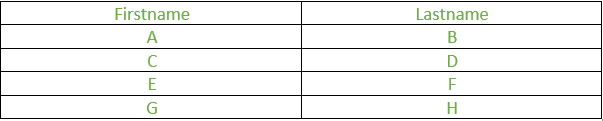# PHP | mysqli_fetch_array() Function

• Last Updated : 23 Apr, 2020

The mysqli_fetch_array() function is used to fetch rows from the database and store them as an array. The array can be fetched as an associative array, as a numeric array or both.

Associative arrays are the arrays where the indexes are the names of the individual columns of the table. On the other hand, numeric arrays are arrays where indexes are numbers, with 0 representing the first column and n-1 representing the last column of an n-column table.

Syntax:

```mysqli_fetch_array ("database_name", "mode")
```

Parameters: This function accepts two parameters as mentioned above and described below:

• database_name: It is the database on which operations are being performed. It is a mandatory parameter.
• mode: It can have three values – MYSQLI_ASSOC, MYSQLI_NUM, and MYSQLI_BOTH. MYSQLI_ASSOC makes the function behave like mysqli_fetch_assoc() function, fetching an associative array, MYSQLI_NUM makes the function behave like mysqli_fetch_row() function, fetching a numeric array while MYSQLI_BOTH stores the data fetched in an array that can be accessed using both column indexes as well as column names.

Program:

 ` ``\$mysqli` `-> query(``\$sql``); ` ` `  `// Numeric array ` `\$row` `= mysqli_fetch_array(``\$conn``, MYSQLI_NUM); ` `printf (``"%s (%s)\n"``, ``\$row``, ``\$row``); ` ` `  `printf(``"\n"``); ` ` `  `// Associative array ` `\$row` `= mysqli_fetch_array(``\$conn``, MYSQLI_ASSOC); ` `printf (``"%s (%s)\n"``, ``\$row``[``"Firstname"``], ``\$row``[``"Lastname"``]); ` ` `  `mysqli_close(``\$conn``); ` `?> `For the above table, the output will be:
Output:

```A    B
C    D
E    F
G    H

A    B
C    D
E    F
G    H
```
My Personal Notes arrow_drop_up
Recommended Articles
Page :